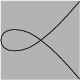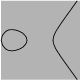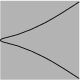Mathematical and Physical Journal
for High Schools
Issued by the MATFUND Foundation
 Already signed up? New to KöMaL?# Exercises and problems in InformaticsMarch 2004

I. 73. Write a program (i73.pas, ...) that determines all prime numbers having N digits with the property that their digits in any order yield another prime number with N digits. The program should display all these primes together with all possible permutations of their digits, but should not display any prime that has already been printed as a permutation of another one. (10 points)

I. 74. Newton studied the following curves described by the equation

y2=x(x2+ax+b).

Write your program (i74.pas) which plots the above curve for any given a and b. The example shows the curve corresponding to a=1, b=0; a=0, b=-1; a=0, b=1, respectively.(10 points)

I. 75. The B(n,k) binomial coefficients in Pascal's triangle can be generalized to negative integer values of n using Pascal's addition formula (that is each element is obtained by adding its upper and upper left neighbours). Prepare your sheet (i75.xls) that computes the negative extension of Pascal's triangle from B(0,0) to B(-n,n), if n is given.

The table shows an example for n=6.

 -6 1 -6 21 -56 126 -252 -5 1 -5 15 -35 70 -126 -4 1 -4 10 -20 35 -56 -3 1 -3 6 -10 15 -21 -2 1 -2 3 -4 5 -6 -1 1 -1 1 -1 1 -1 0 1 0 0 0 0 0

(10 points)